# Optics Flashcards Preview

## AP Physics > Optics > Flashcards

Flashcards in Optics Deck (62):
1

## What is the law that governs the reflection of a light ray off a flat surface?

### θi = θrThe law of reflection states that: light incident on a reflective surface at some angle will bounce off at an equal angle of reflection, as measured from the normal.2

## What will the angle of reflection be for a light ray incident on a surface, making an angle of 35 degrees to the surface?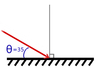### θr = 55 degrees Remember: the angles of incidence and reflection must be measured relative to the surface's normal. 90 - 35 = 55. θi and θr are shown below.3

## refraction

### Refraction is when a light ray crosses a boundary from one transparent medium to another. When the light ray changes media it bends either towards or away from the normal, depending on the properties of the respective media.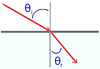4

5

6

## How is the path of a light ray affected when the ray crosses a boundary from air (low n) into glass (high n)?### When a light ray crosses from air into glass, it bends towards the normal of the surface once inside the glass. Any boundary crossing into a medium with a higher index of refraction will cause light to bend towards the normal.7

8

## Snell's law

### Snell's law gives the direct relationship between angles of incidence and refraction, from a light ray crossing the boundary between media: n1sin(θ1) = n2sin(θ2) where n1 = index of refraction on the incident side θ1 = angle of incidence n2 = index of refraction on the refraction side θ2 = angle of refraction9

## critical angle θc

### The critical angle θc is the angle of incidence in one medium at which the angle of refraction into the new medium would become 90 degrees or higher. There is only a critical angle for refraction across a boundary at which the index of refraction decreases, such as glass into air.10

11

## total internal reflection

### When a light ray approaches a boundary of decreasing index of refraction at an angle of incidence greater than the critical angle, it undergoes total internal reflection. The light ray entirely reflects, staying in the incident medium. No light refracts across the boundary.12

13

14

## Why is a beam of sunlight separated into a rainbow of different colors as it passes through a prism?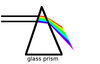### The rainbow occurs due to dispersion. The white sunlight beam is made up of all the colors of visible light mixed together, as is all white light. The glass of the prism is dispersive, and different colors of light refract differently as they pass through the prism, so they are separated upon exiting the back surface. Blue light is refracted more than red light in glass.15

## When white light is separated by a prism into its component colors, in what order do they appear?

### The separated colors are, in order, red, orange, yellow, green, blue, indigo, violet. This is easily remembered by the mnemonic ROYGBIV (Roy Gee Biv).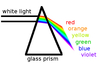16

## the focal point of an optic

### An optic's focal point is the location where all rays parallel to the principal axis of the optic will cross after reflecting from/refracting through the optic. An optic with a short focal point is a strong optic, bending light severely, while an optic with a long focal point is a weak optic, affecting light rays less significantly.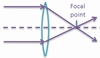17

18

## What are the characteristics of the parallel ray in an optical system?

### The parallel ray is a light ray which reflects off the top of an object, and travels parallel to the principal axis of the optic. After reflecting off/refracting through the optic, the parallel ray crosses the principal axis at the focal point, and can be used to help locate the image.19

## What are the characteristics of  the focal ray in an optical system?

### The focal ray is a light ray which reflects off the top of an object, and travels through the focal point of the optic. After reflecting off/refracting through the optic, the focal ray emerges parallel to the principal axis of the optic, and can be used to help locate the image.20

## What are the characteristics of the center ray in an optical system?

### The center ray is a light ray which reflects off the top of an object, and impacts the optic directly in the center. The center ray is undeflected by reflecting off/refracting through the optic. It travels in a straight line, and can be used to help locate the image.21

## What makes a mirror converging?

### A mirror is converging if any inbound light ray which is parallel to the mirror's principal axis crosses the axis after reflecting off the mirror. Converging mirrors are concave in shape.22

## What is the radius of curvature of a converging mirror?

### The radius of curvature of a mirror is the radius of the sphere of which the mirror is a small portion.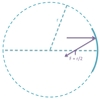23

## How does the radius of curvature of a converging mirror compare to the mirror's focal length?

### For all spherical mirrors, the focal length is one-half the radius of curvature.24

25

26

## An optical system consists of an object and a single converging mirror. If the object is a distance between f and 2f from the optic, what are the properties of the final image?### The image is further from the optic than the object is, and the image is real, magnified, and inverted.  Find where the parallel, focal, and center rays cross after reflecting off the mirror to locate the image. Since the rays cross after reflection, the image is real.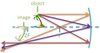27

## An optical system consists of an object and a single converging mirror. If the object is at the focal length f, what are the properties of the final image?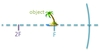### No image is formed. The parallel and center rays are parallel to one another after reflecting off the mirror. Since the rays never cross after reflection, no image is formed.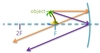28

## An optical system consists of an object and a single converging mirror. If the object is inside the focal length f, what are the properties of the final image?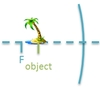### The image is further from the optic than the object is, and the image is virtual, magnified, and upright.  The parallel and center rays are diverging after reflecting off the mirror. To locate the image, the rays much be projected behind the mirror to the point where they cross. Since only the virtual rays cross, the image is virtual.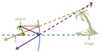29

30

## What is the focal length of a converging mirror?

### The focal length is the distance from the mirror to the focal point. Rays parallel to the optical axis cross at the focal point after reflecting off the mirror. The focal length of any spherical optic is one-half its radius of curvature.31

32

33

34

## For an optical system, if the object and image distance are o = 30 cm and i = 60 cm, respectively, what is the magnification of the image?

### m = -2 Remember, m = -i/o; so for this system: m = -i/o = -(60/30) = -2 So the image is inverted, and twice as tall as the object.35

## For an optical system, if the object and image distance are o = 20 cm and i = -10 cm, respectively, what is the magnification of the image?

### m = +½ Remember, m = -i/o; so for this system: m = -i/o = -((-10)/20) = +½ So the image is upright, and half as tall as the object.36

## What makes a mirror diverging?

### A mirror is diverging if any inbound light ray which is parallel to the principal axis points away from the axis after reflecting off the mirror. All diverging mirrors are convex in shape.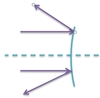37

38

## For an optical system made up of a single mirror, if the object distance is o = 30 cm and the image distance is i = -30 cm, what type is the mirror?

### f = infinity, and the mirror is planar.Given i and o, f can be calculated as:1/f = 1/o + 1/i = 1/30 + 1/(-30) =  0 f = infinityPlanar mirrors produce virtual images, and i = -o.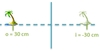39

## For an optical system made up of a single mirror, if the object distance is o = 15 cm and the image distance is i = 30 cm, what type is the mirror?

### f =  10 cm, and the mirror is converging. Given i and o, f can be calculated as: 1/f = 1/o + 1/i = 1/15 + 1/30 =  3/30 f = 30/3 = 10 cm40

## What is the radius of curvature of a diverging mirror?

### The radius of curvature of a diverging mirror is the radius of the sphere of which the mirror is a small portion.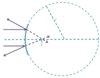41

## How does the radius of curvature of a diverging mirror compare to the mirror's focal length?

### For all spherical mirrors, the focal length is one-half the radius of curvature.42

## What is the focal length of a diverging mirror?

### The focal length is the distance from the mirror to the focal point. Virtual extensions of rays parallel to the principal axis cross at the focal point after reflecting off the mirror. The focal length of any spherical optic is one-half its radius of curvature.43

## An optical system consists of an object and a single diverging mirror. What are the properties of the final image?### The image is closer to the optic than the object is, and the image is virtual, reduced, and upright.  Virtual extensions of the parallel and center rays cross behind the mirror after reflection; this is where the image is located. Since only the virtual rays cross after reflection, the image is virtual.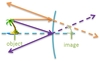44

45

## What makes a lens converging?

### A lens is converging if any inbound light ray which is parallel to the optical axis crosses the axis after refracting through the lens. Converging lenses are convex in shape.46

## What is the focal length of a converging lens?

### The focal length is the distance from the lens to the focal point. Rays parallel to the principal axis cross at the focal point after refracting through the lens.47

## An optical system consists of an object and a single converging lens. If the object is a distance between f and 2f from the optic, where are the properties of the final image?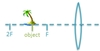### The image is further from the optic than the object is, and the image is real, magnified, and inverted.  Find where the parallel, focal, and center rays cross after refracting through the lens to locate the image. Since the rays cross after refraction, the image is real.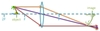48

## An optical system consists of an object and a single converging lens. If the object is at the focal length f, what are the properties of the final image?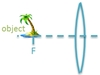### No image is formed. The parallel and center rays are parallel to one another after refracting through the lens. Since the rays never cross after refraction, no image is formed.49

## An optical system consists of an object and a single converging lens. If the object is inside the focal length f, what are the properties of the final image?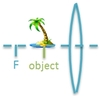### The image is further from the optic than the object is, and the image is virtual, magnified, and upright.  The parallel and center rays are diverging after refracting through the lens. To locate the image, the rays much be projected behind the lens to the point where they cross. Since only the virtual rays cross, the image is virtual.50

51

## What makes a lens diverging?

### A lens is diverging if any inbound light ray which is parallel to the principal axis points away from the axis after refracting through the lens. Diverging lenses are concave in shape.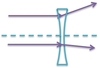52

## What is the focal length of a diverging lens?

### The focal length is the distance from the lens to the focal point. Virtual extensions of rays parallel to the principal axis cross at the focal point after refracting through the mirror.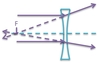53

## An optical system consists of an object and a single diverging lens. What are the properties of the final image?### The image is closer to the optic than the object is, and the image is virtual, reduced, and upright.  Virtual extensions of the parallel and center rays cross behind the lens after refraction; this is where the image is located. Since only the virtual rays cross after refraction, the image is virtual.54

55

56

57

58

## chromatic aberration of a lens

### Chromatic aberration is the characteristic colored halo around an image created by a lens. This occurs due to dispersion. Different colors of light will focus at different points, so an image created by a lens of an object illuminated by white light will appear blurred.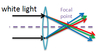59

## spherical aberration of an optic

### Spherical aberration is the characteristic blurriness of an image created by a real spherical lens. Spherical optics focus light rays well, so long as the light rays are near the center of the optic. Light rays near the optic's edge, however, will not focus at exactly the same point, so images including light rays from the optic's edge will appear blurred.60

61

62